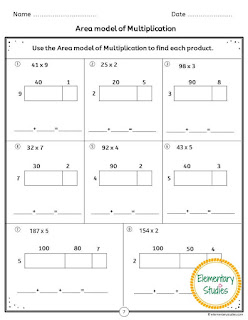## Elementary Studies

### Multi-digit Multiplication

This is the third packet in my Multiplication product series. The initial two were Multiplication Facts and Multiplication Properties. Knowing the Multiplication facts and the Multiplication properties are important pre-requisites for learning multi digit multiplication.

Learning multi-digit multiplication require lots of practice. In this multi digit multiplication packet, I have included the practice sheets using different strategies, including the Standard Algorithm, Expanded Algorithm, Distributive properties, Area Model and Estimates to multiply up to a four-digit number by a one-digit number.Happy Teaching!

Shimps